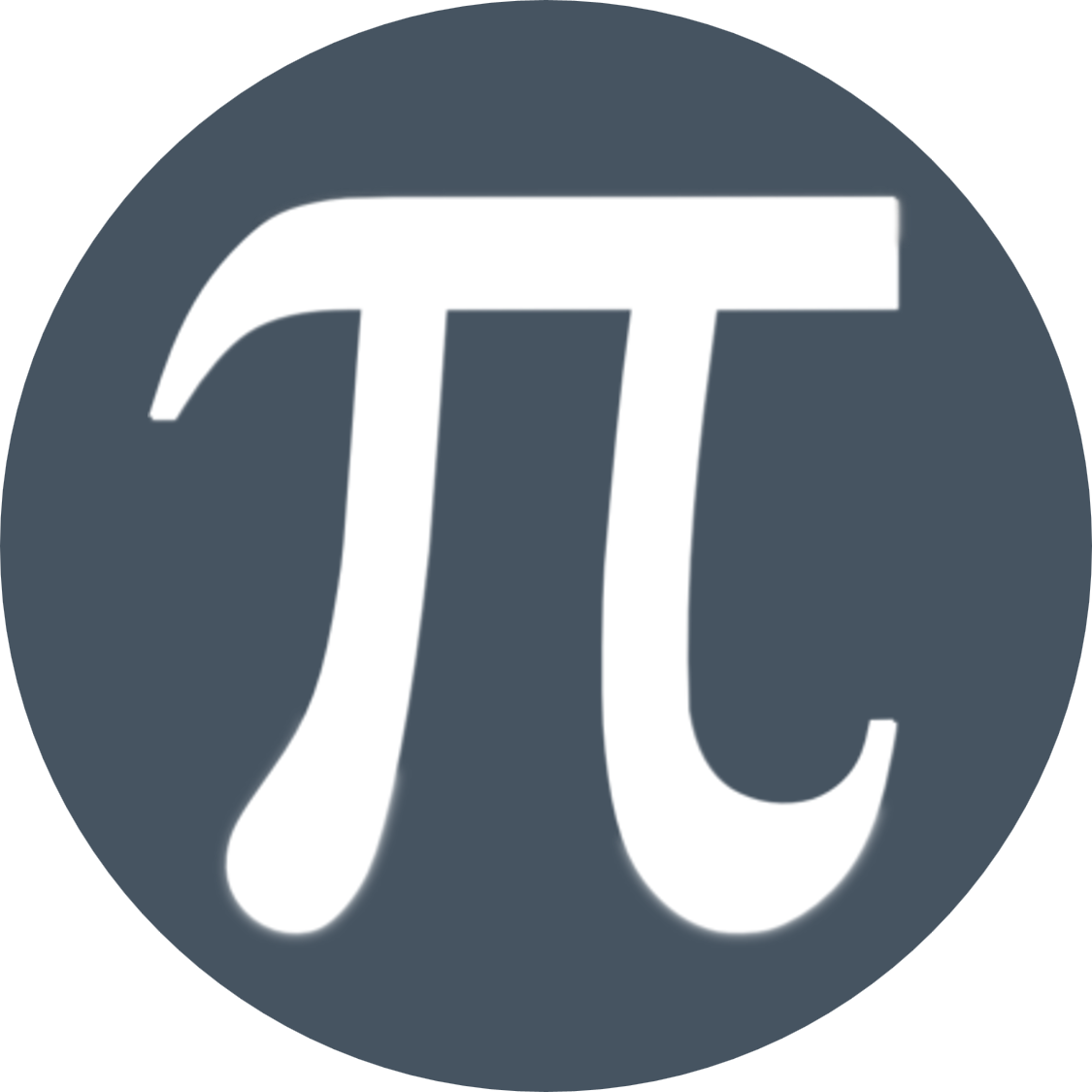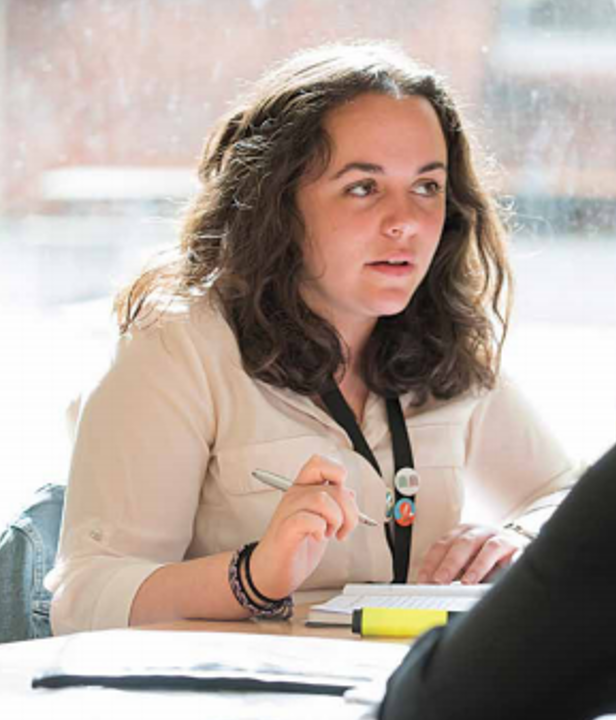# Maths - Core

If you would like to view this content please

If you would like to view this content please

## Which Maths Course should I choose?

CHOOSE A LEVEL MATHEMATICS

• Did you enjoy the challenge of Algebra and Trigonometry in GCSE Maths and want to extend and develop your knowledge of mathematical concepts and techniques?
• Do you want to study a well-respected A level with numerous desirable and transferable skills?
• Do you want a solid base from which to support your study of Science Technology or Engineering subjects?

CHOOSE LEVEL 3 MATHEMATICAL STUDIES (Core Maths)

• Did you find the A level Mathematics preparation too much of a challenge?
• Enjoyed GCSE Maths and want tocontinue your studies but also have chosen 3 other A level subjects
• Want, or need, more mathematics to support your other studies in Geography, Biology, Chemistry, Product Design, Psychology, PE etc.?

CHOOSE A LEVEL FURTHER MATHS

• Are you already studying A level Mathematics and want a 4th challenging A level?
• Are you able to understand difficult maths concepts quickly?
• Do you plan to study Mathematics at University or like the idea of studying Physics or Computing at a top University?

Please also read the Mathematics at NDHS Sixth Form document for further detail.

###Why study Maths - Core?

This exciting new subject is for students who are studying with a Mathematical elements, for example; Biology, Economics and Psychology.  All of these subjects contain a level of data analysis.  The certificate is not a full A-Level and is only available as an addition to a 3 A-Level package.

The Level 3 Mathematical Studies (Core Maths) qualification will consolidate in applying your mathematical understanding, building confidence and competence in applying mathematical techniques to solve a range of problems, as well as introducing new techniques and concepts in preparation for further study and future employment within a broad range of academic, professional and technical fields.

Students will develop mathematical modelling, evaluation and reasoning skills to solve problems which will not be well defines and may not have a unique solution.  You will be expected to develop your understanding, communication and enjoyment of mathematics through this course.## Where will this subject lead me?

Mathematical Studies aims to prepare students for the mathematical demands of higher education and work where there is a distinct mathematical or statistical element, but where the mathematical demands do not stretch to requirement for A-Level Mathematics.

Mathematics is a well-respected and sought after qualification for a wide verity of Higher Education Courses; it is essential for a degree in mathematics or physics.  Logical thinking and problems solving skills are highly valued in the work place and lucrative industries like IT, banking and the stock market need people confident in using mathematics on a daily basis.

## Maths FAQs

Why is studying A Level Maths useful?

A Level maths is an interesting and rewarding course which extends the topics you learnt at GCSE and applies these topics to mechanics and statistics. Through taking A Level maths, you will enhance your numeracy, logic and reasoning skills plus the problem solving work that you will do will help you to think creatively. All of these skills are highly valued by employers and universities which is why A Level Maths is such a useful course. There is currently a huge demand from employers for STEM students and A Level Maths is often a requirement for most university courses in these fields.

What skills will I need to be able to do A Level maths?

You will need a minimum of grade 6 GCSE to study A Level maths. The most important GCSE topic that you will need for A Level maths is algebra since it will be used in nearly every A Level maths topic. It's very important that you are comfortable with GCSE algebra before beginning the A Level course.

The workload is high for maths since you will need to understand a topic as well as attempt lots of questions. But if you enjoy a certain topic or find it easy, you may be able to plough through a set of questions and the workload may seem low compared to other subjects. But on the other hand, if you find the topic challenging then attempting 20 questions on this topic may take up a lot of your time. Comparing A Level to GCSE, most students will say that the difficulty level very quickly increases and the second year of the course will be a step up again from the first year.

What topics will I study?

The content is the same across all examination boards and is divided into pure maths and applied maths which includes statistics and mechanics. The topics that you will learn are shown below: Pure mathematics (2/3 of the content) : Algebra and functions, proof, coordinate geometry, sequences and series, trigonometry, logarithms, calculus, numerical methods, vectors Statistics (1/6 of the content) : Sampling, data representation, probability, probability distributions, hypothesis testing Mechanics (1/6 of the content) : Mechanical quantities and units, kinematics, forces and Newton's laws, moments Exam boards provide a "large data set" which you will work with throughout the statistics course and you will need to be familiar with it for the final exam.

How will it be assessed?

For the new linear course, the A Level will be assessed at the end of your course (normally at the end of the second year) and is 100% exam with no coursework. The exams will assess everything that you have learnt in pure maths, mechanics and statistics and will be graded from A* to E.

Will not taking A Level Physics be a disadvantage?

The general consensus is that studying mechanics as part of the maths course will help you with physics but the physics course won't help much with mechanics. While you will need to understand some physical concepts, in maths the focus is more on the applications of pure maths to physics questions. So you don't need to worry if your classmates are studying physics and you are not.

What is Core Maths?

Core Maths is a course that develops the application of mathematical techniques in context often relating to more real-world applications. It is an option for students who do not want to take a full A level in mathematics but would benefit from continued study in maths to support their other A level subjects. It is equivalent in size to an AS level and would gain equivalent UCAS points to an AS level. Core maths will be graded A to E in parallel to the AS qualifications. At Notre Dame Core maths can only be chosen in addition to 3 other A level subjects.

Should I study Further Mathematics?

Further maths is significantly more challenging than A level maths and requires a minimum of grade 7 GCSE. Further Maths can only be taken in addition to level maths, it is designed to stretch and challenge able mathematicians and prepare them for university courses in mathematics as well as other scientific and engineering subjects. Careful research is advised where possible to decide if further maths will be a requirement of any university course.

How will Further Mathematics assessed?

For the new linear course, the A Level will be assessed at the end of your course (normally at the end of the second year) and is 100% exam with no coursework. The exams will assess everything that you have learnt in pure maths, mechanics and discrete maths and will be graded from A* to E.

## Contact a Teacher

If you have any subject specific questions that you'd like to ask.  You are very welcome to contact the relevant Head of Subject.  To find their email address click on Teaching Staff.  For general enquiries, contact the 6th Form Team.

Core Maths Induction Resources